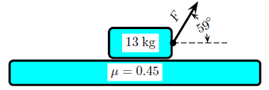# Problem: At the beginning of a new school term, a student moves a box of books by attaching a rope to the box and pulling with a force of F = 85.7 N at an angle of 59°, as shown in the figure. The acceleration of gravity is 9.8 m/s2. The box of books has a mass of 13 kg and the coefficient of kinetic friction between the bottom of the box and the floor is 0.45. What is the acceleration of the box?1. 1.528112. 1.14163. 2.071824. 1.277615. 1.238786. 1.40247. 1.838568. 1.049069. 1.6525410. 1.43878

🤓 Based on our data, we think this question is relevant for Professor Hoxha's class at HUNTER.

###### Problem Details

At the beginning of a new school term, a student moves a box of books by attaching a rope to the box and pulling with a force of F = 85.7 N at an angle of 59°, as shown in the figure. The acceleration of gravity is 9.8 m/s2. The box of books has a mass of 13 kg and the coefficient of kinetic friction between the bottom of the box and the floor is 0.45. What is the acceleration of the box?

1. 1.52811

2. 1.1416

3. 2.07182

4. 1.27761

5. 1.23878

6. 1.4024

7. 1.83856

8. 1.04906

9. 1.65254

10. 1.43878